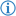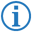The equal operator is a type of comparison operator which returns the Boolean value `true` if the number objects in input are equal, else it returns `false`.

Its token is named EQUAL.

## FDS### Example

`If`` ``Spike's age`` ``was`` ``10`` ``then``:``...`

This executes the next part of code if the variable `Spike's age` is equal to `10`.

This is equivalent to the following Java code:

```if(SpikesAge == 10) { ...
```